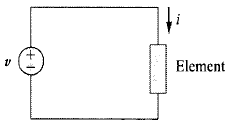Problem

# In Fig. 6.43, if i = cos 4t and v = sin 4t, the element is:(a)a resistor(b) a capacitor(c)...

In Fig. 6.43, if i = cos 4t and v = sin 4t, the element is:

(a)a resistor

(b) a capacitor

(c) an inductorFigure 6.43

#### Step-by-Step Solution

Solution 1

Refer to Figure $$6.43$$ in the textbook.

Write the expression for voltage across the element.

$$v=\sin 4 t$$

Write the expression for current through the element.

$$i=\cos 4 t$$

From the trigonometric properties:

\begin{aligned} &\cos x=\sin \left(x+90^{\circ}\right) \\ &\cos (4 t)=\sin \left(4 t+90^{\circ}\right) \\ &i=\sin \left(4 t+90^{\circ}\right) \end{aligned}

Write the expression for current through the element.

$$i=\sin \left(4 t+90^{\circ}\right)$$

Write the expression for voltage across the element.

$$v=\sin 4 t$$

The impedance of resistor is pure real value and it does not have any phase variation that is the phase difference between voltage and current should be zero. In inductors, the current lags the voltage by $$90^{\circ}$$ and in capacitors, the current leads the voltage by $$90^{\circ}$$.

Here, the current is leading the voltage by $$90^{\circ}$$. This phenomenon occurs only in capacitors. Hence, the element should be a capacitor.